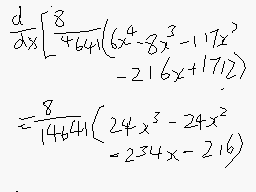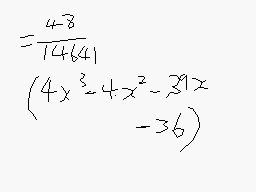# Continuous Random Variable question

• PuzzledMe

## Homework Statement

A train is scheduled to arrive at the station at 0820 hours every morning and the amount of minutes that the train arrives after 0820 hours is denoted by X. The probability that the train arrives more than x minutes after 0820 hours is given by

{ 1 for x ≤ [(-3)/2],
{ (8/14641) [6(x^4) - 8x³ - 117(x²) - 216x + k) for [(-3)/2] ≤ x ≤ 4,
{ 0 for x ≥ 4,

where k is a constant.

Show that,

1) k = 1712
ii) The probability density function, f, of X is f(x) = (48/14641) (3 + 2x)²(4 - x) for [(-3)/2] ≤ x ≤ 4, and sketch the graph of f.
iii) the most probable arrival time of the train is 10 seconds after 0822 hours.

Find the probability that the train arrives before 0820 hours.

Problem statement is underlined. Having problems to prove this.

## Homework Equations

F(x) = ∫ f(x) dx
Question relating to cumulative distributive function. Part ii requiring to relate cumulative distributive function to probability density function.

## The Attempt at a SolutionWhy I'm stuck =>
(4x³ - 4x² - 39x - 36) ≠ (3 + 2x)²(4 - x)
(3 + 2x)²(4 - x) = (-4x³) - 4x² - 39x + 36

Stuck after that as the positive and negative signs seems swapped. Checked again and nothing seems to change.

Is this due to a faulty question or I'm missing out something vital?

Last edited:

Why I'm stuck =>
(4x³ - 4x² - 39x - 36) ≠ (3 + 2x)²(4 - x)
(3 + 2x)²(4 - x) = (-4x³) - 4x² - 39x + 36

Stuck after that as the positive and negative signs seems swapped. Checked again and nothing seems to change.

Hi PuzzledMe!You can check which is right by putting x = 4: that should give 0.

(4x³ - 4x² - 39x - 36) = 256 - 64 - 156 - 36 = 0
(-4x³) - 4x² - 39x + 36 = -256 -64 -156 + 36 ≠ 0.

So your expansion of (3 + 2x)²(4 - x) must be wrong!

And it is … try again!Thanks tim for the tireless response, I've solved it from here, sorry about the late reply but I owe you one!